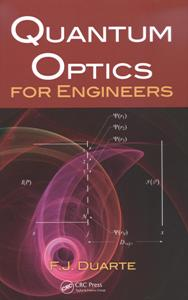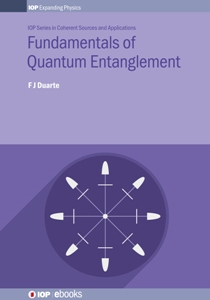# Quantum Entanglement Engineering and Applications

## F. J. Duarte and T. S. Taylor, Quantum Entanglement Engineering and Applications (Institute of Physics, Bristol, 2021)

ISBN: 978-0-7503-3405-1

10 chapters, 1 appendix, 72 figures, 79 problems, approximately 860 equations, in 200 pages.Quantum Entanglement Engineering and Applications (Institute of Physics, Bristol, 2021) at Amazon

Quantum Entanglement Engineering and Applications (Institute of Physics, Bristol, 2021) at IoP

Watch interview on Quantum Entanglement Engineering and Applications in youtube (English)

Watch interview on Quantum Entanglement Engineering and Applications in youtube (Spanish)

Article on Quantum Entanglement Engineering and Applications at Physics World

## CONTENTS

### 1.Introduction

1.1 Introduction

1.2 Essentials of quantum mechanics

1.2.1 The quantum photon

1.2.2 Planckĺs constant: h

1.3 Wardĺs perspectives

1.4 The philosophy and the physics of quantum entanglement

1.4.1 The philosophy

1.4.2 The physics

1.5 Quantum entanglement as a discipline

1.6 Quantum entanglement engineering and applications

1.7 Intent

Problems

References

### 2. Diracĺs notation for quantum entanglment

2.1 Introduction

2.2 Diracĺs bra ket notation

2.3 Diracĺs notation in N-slit interferometers

2.4 Semi coherent interference

2.5 Expanded series of N-slit quantum interference probabilities

2.6 From quantum probabilities to measurable intensities

2.7 Dirac identities

2.8 Quantum entanglement probability amplitudes for n = N = 2

2.9 Quantum entanglement probability amplitudes for n = N = 4

2.10 Quantum entanglement probability amplitudes for n = N = 8

2.11 Quantum entanglement probability amplitudes for n = N = 16

2.12 Quantum entanglement probability amplitudes for n = N = 21,22 ,23,24...2r

2.13 Quantum entanglement probability amplitudes for n = N = 3

2.14 Quantum entanglement probability amplitudes for n = N = 6

2.15 Beyond single quanta-pair quantum entanglement

2.16 Discussion

Problems

References

### 3. Indistinguishability

3.1 Introduction

3.2 Indistinguishability in quantum interference

3.3 Indistinguishability in Diracĺs identities

3.4 Indistinguishability in quantum entanglement

3.5 Indistinguishability in quantum ensembles

3.6 Discussion

Problems

References

### 4.Quantum interferometry via Diracĺs bra ket notation

4.1 Introduction

4.2 The N-slit interferometer

4.3 Interferometers configured by beam splitters

4.3.1 The single beam splitter

4.3.2 The Mach-Zehnder interferometer

4.3.3 The Michelson interferometer

4.3.4 The Sagnac interferometer

4.4 Beam splitter matrices and Diracĺs bra ket notation

4.4.1 The beam splitter and the Hadamard gate

4.5 Revisiting the single beam splitter

Problems

References

### 5. Vectors, matrices, and tensors for quantum entanglement

5.1 Introduction

5.2 Vector basics

5.3 Vector products

5.3.1 Dot product

5.3.2 Cross product

5.3.3 The ket bra product

5.3.4 Vector direct product

5.3.5 Vector outer product

5.4 Matrix algebra

5.4.1 The identity matrix

5.4.2 The inverse matrix

5.4.3 Matrix determinant and trace

5.4.4 Eigenvalues and eigenvectors

5.5 The Pauli matrices

5.5.1 Eigenvalues of the Pauli matrices

5.6 Unitary matrices

5.7 The tensor product

Problems

References

### 6. Five avenues to the probability amplitude of quantum entanglement

6.1 Introduction

6.2 Wardĺs heuristic derivation

6.2.1 The quantum entanglement probability

6.3 Quantum entanglement from Feynmanĺs two state approach

6.4 Quantum entanglement from N-slit interference

6.5 Quantum entanglement from the Pauli matrices

6.6 Quantum entanglement from the Hadamard gate

6.7 Quantum interference or quantum entanglement?

Problems

References

### 7. Quantum entanglement in matrix notation

7.1 Introduction

7.2 Quantum entanglement probability amplitudes

7.3 From ket vectors to polarization matrices

7.4 The Pauli matrices and quantum entanglement

7.5.1 Derivation of the matrix for the Hadamard gate

7.6 Optical matrices based on the probability amplitudes of quantum entanglement

7.7 Polarization rotators for quantum entanglement

7.7.1 Wave plates

7.7.2 Rhombois

7.7.3 Prismatic rotators

7.8 Quantum operations with polarization rotators

7.9 Quantum operations with the Hadamard gate

Problems

References

### 8. Quantum entanglement applications

8.1 Introduction

8.2 Classical cryptography concepts

8.3 Quantum entanglement applications to cryptography

8.3.1 Quantum key distribution (QKD)

8.3.2 Experiments

8.3.3 All-quantum security protocol

8.4 Quantum entanglement applications to teleportation

8.4.1 Mathematical methodology

8.4.2 Technology

8.5 Quantum computing

8.51 The Pauli gates

8.5.2 The Pauli-X gate and quantum entanglement

8.5.3 The Hadamard gate and quantum entanglement

8.5.4 Technology

8.6 Quantum entanglement applications to metrology

8.7 Overview

Problems

References

### 9. Space-to-space quantum communications

9.1 Introduction

9.2 Relevant satellite engineering parameters

9.3 Beam divergence

9.4 Optical configuration for quantum satellite communications

9.4.1 Satellite-satellite

9.4.2 Earth-space-Earth

9.5 Existing data from experiments on quantum satellite communications

9.5.1 The Delingha-Lijiang experiment

9.5.2 The International Space Station experiment

9.6 Satellite networks and their dependence on entangled photon source characteristics

9.6.1 The quantum repeater and quantum data teleportation

9.7 Sources for quantum entanglement communications

9.8 Outlook

Problems

References

### 10. Quantum entanglement and the interpretations of quantum mechanics

10.1 Introduction

10.2 Many alternative interpretations

10.3 Guidance from quantum titans

10.3.1 Dirac

10.3.2 Feynman

10.3.3 Dyson

10.3.4 Lamb

10.3.5 Ward

10.4 Hidden variable theories

10.4.1 Bohmĺs hidden variable theories

10.4.2 Bellĺs theorem

10.4.3 Comment on the EPR paper

10.5 A pragmatic perspective on the interpretations of quantum mechanics

10.6 Quantum principles

10.7 Quantum measurements

10.7.1 Dysonĺs first layer quantum mechanics

10.7.2 Dysonĺs second layer quantum mechanics

10.7.2.1 Time flow

10.7.3 Quantum measurement sequence

10.7.4 Quantum entanglement measurements

10.7.5 The observer

10.7.6 On Ĺthe collapse of the wave functionĺ

10.8 Is quantum entanglement the essence of quantum mechanics?

10.9 On the origin of the Dirac-Feynman principle

10.10 Quantum pragmatism free of paradoxes

Problems

References

## Appendix

### A. More on Diracĺs notation: application to laser cavities and interference

A.1 Introduction

A.2 The cavity linewidth equation: a heuristic approach

A.3 The cavity linewidth equation: an interferometric approach

A.4 Coherent electrically-pumped organic interferometric emitters

A.4.1 Coherence

A.4.2 The quantum perspective

A.5 Quantum interference to classical interference

A.5.1 Classical interference

A.5.2 Quantum interference

A.6 Intracavity double-mode beating instability

A.6.1 Alternative double-mode instability characterization

A.7 Diracĺs Ĺcoincidencesĺ

Problems

ReferencesPage published on the 20th of September, 2021

Updated on the 20th of September, 2019